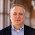## Sunday, June 24, 2018

### Statistics Sunday: Converting Between Effect Sizes for Meta-Analysis

Converting Between Effect Sizes I'm currently working on my promised video on mixed effects meta-analysis, and was planning on covering this particular topic in that video - converting between effect sizes. But I decided to do this as a separate post that I can reference in the video, which I hope to post next week.

As a brief refresher, meta-analysis is aimed at estimating the true effect (or effects) in an area of study by combining findings from multiple studies on that topic. Effect sizes, the most frequently used being Cohen's d, Pearson's r, and log odds ratio, are estimated from information in study reports and presentations. There's a lot of variation in how clearly reports and documents describe the findings and the information given to estimate the study's overall effect. But when you conduct the meta-analysis, whether using fixed, random, or mixed effects analysis, you need to use only one type of effect size. That means that, sometimes, studies will give you a different type of effect size than you plan to use. Fortunately, there are ways to convert between effect sizes and use different types of statistical information to generate your estimates.

First up, converting between those key effect sizes. In the meta-analysis I performed in grad school, I examined the effect of pretrial publicity on guilt. There are two ways guilt was frequently operationalized in the studies: as a guilty/not guilty verdict or as a continuous guilt rating. For those outcomes, we would likely use, respectively, log odds ratio and Cohen's d. The escalc function in the metafor package can compute log odds ratio for guilty/not guilty counts, and Cohen's d for mean and standard deviation of the guilt ratings. But studies may use different types of information when presenting their results, so you may not be able to simply compute those effect sizes.

For instance, a study using verdict may present a chi-square and one of its effect sizes, Cramer's V, which is very similar to a correlation coefficient. How can I convert that into log odds ratio?

To convert from one effect size to the other, you need to follow a prescribed path, which can be seen in the diagram below. What this diagram tells you is which effect sizes you can convert between directly: you can directly convert between log odds ratio and Cohen's d, and between Cohen's d and Pearson's r. If you wanted to convert between Pearson's r and log odds ratio, you'll first need to convert to Cohen's d. You'll need to do the same thing for variance - compute it for the native effect size metric, then convert that to the new effect size metric.

Let's start by setting up functions that will convert between our effect sizes for us, beginning with Cohen's d and log odds ratio. Then we'll demonstrate with some real data.

```#Convert log odds ratio to d
ltod <- function(lor) {
d = lor * (sqrt(3)/pi)
return(d)
}
vltovd <- function(vl) {
vd = vl * (3/pi^2)
return(vd)
}

#Convert d to log odds ratio
dtol <- function(d) {
lor = d*(pi/sqrt(3))
return(lor)
}
vdtovl <- function(vd) {
vl = vd*(pi^2/3)
return(vl)
}
```

You'll notice a mathematical symmetry in these equations - the numerators and denominators switch between the equations. Now let's set up equations to r and d. These equations are slightly more complex and will require a few additional arguments. For instance, converting the variance of r to variance of d requires both the variance of r and r itself. Converting from d to r requires group sample sizes, referred to as n1 and n2.

```#Convert r to d
rtod <- function(r) {
d = (2*r)/(sqrt(1-r^2))
return(d)
}
vrtovd <- function(vr,r) {
vd = (4*vr)/(1-r^2)^3
return(vd)
}

#Convert d to r
dtor <- function(n1,n2,d) {
a = (n1+n2)^2/(n1*n2)
r = d/(sqrt(d^2+a))
return(r)
}
vdtovr <- function(n1,n2,vd,d) {
a = (n1+n2)^2/(n1*n2)
vr = a^2*vd/(d^2+a)^3
return(vr)
}
```

Remember that the metafor package can compute effect sizes and variances for you, so you might want to run the escalc on the native effect sizes so that you have the estimates and variances you need to run these functions. But if you ever find yourself having to compute those variances by hand, here are the equations, which we'll use in the next step.

```vard <- function(n1,n2,d) {
vd = ((n1+n2)/(n1*n2)) + (d^2/(2*(n1+n2)))
return(vd)
}

varr <- function(r,n) {
vr = (1-r^2)^2/(n-1)
return(vr)
}

varlor <- function(a,b,c,d) {
vl = (1/a)+(1/b)+(1/c)+(1/d)
return(vl)
}
```

One of the studies I included in my meta-analysis gave Cramer's V. It had a sample size of 42, with 21 people in each group. I'd like to convert that effect size to log odds ratio. Here's how I could do it.

```cramerv <- 0.67
studyd <- rtod(cramerv)
studyvr <- varr(0.67,42)
studyvd <- vrtovd(studyvr,cramerv)
dtol(studyd)
```
```##  3.274001
```
```vdtovl(studyvd)
```
```##  0.5824038
```

I can now include this study in my meta-analysis of log odds ratios.

What if my study gives different information? For instance, it might have given me a chi-square or a t-value. This online effect size calculator, created by David Wilson, coauthor of Practical Meta-Analysis, can compute effect sizes for you from many different types of information. In fact, spoiler alert: I used an earlier version of this calculator extensively for my meta-analysis. Note that this calculator returns odds ratios, so you'll need to convert those values into a log odds ratio.

1.2.